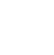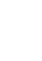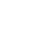# Chemistry Paper 2 Questions and Answers - Form 4 Opener Term 1 Exams 2022

CHEMISTRY
PAPER 2

Instructions

• Attempt all the questions in the spaces provided.

Questions

1. The diagram below shows chromatograms for five different dyes.1. Name the technique used to separate the dyes. (1 mk)
2. What conditions are required to separate the chromatograms present in a dye? (2 mks)
3. What is meant by the term solvent front? Indicate its position in the diagram. (1 ½ mks)
4. Which chromatogram were present in dye D. (1 mk)
5. Which dye is insoluble? (1 mk)
6. Which dye is pure? Explain. (1 ½ mks)
7. Which chromatogram is most soluble? (1 mk)
8. How can one obtain the extract of the blue dye? (2 mks)
9. The results showed that dye E contained unwanted colour. Identify the colour. (2 mks)
2. Air was passed through several reagents as shown in the flow chart below.1. Write an equation for the reaction which takes place in the chamber with.
1. Concentrated Sodium hydroxide. (1 mk)
2. Excess heated copper turnings. (1 mk)
3. Excess heated magnesium (1 mk)
4. Name one gas which escapes from the chamber containing magnesium powder. Give a reason for your answer. (2 mks)
2. The diagram below shows an experimental set up for the laboratory preparation of nitrogen gas.1. Name the reagent B and state its role. (2 mks)
2. What is the observation made in the combustion tube? (1 mk)
3. Nitrogen gas collected using this method is not pure. Explain. (1 mk)
4. Give a reason why liquid nitrogen is used for storage of semen for artificial insemination. (1 mk)
3. Use the grid below to answer the questions that follow. The letters do not represent actual symbols of the elements.1.
1. Which element form ions with a charge of -2? Explain. (2 mks)
2. Identify and explain the elements with great tendency of forming covalent compound. (2 mks)
2. How do the reactivity of the following elements compare? Explain.
1. T and V (2 mks)
2. T and E (2 mks)
3. S and Z (2 mks)
4. Select the element with the largest atomic radius. Give a reason. (2 mks)
4. Study the flowchart below and answer the questions that follow.1. Identify substances A, B, C and E. (2 mks)
2. Identify process X and Y. (1 mk)
3. Write chemical equations to show how substance E may be obtained from B. (2 mks)
4. Draw the structure of; (2 mks)
1. Polymer G
2. The repeating unit of polymer G
5. A polymer is represented by:1. Draw the structure of the monomer. (1 mk)
2. A sample of the polymer was found to have relative molecular mass of 3952. Determine the volume of n. (H = 1.0, C = 12.0) (2 mks)
3. State one demerits of this kind of polymer as a synthetic fibre. (1 mk)
5. The diagram below shows the extraction of Sulphur by the Frasch process.1. State what passes through pipe 1 and 3. (2 mks)
2. Give the purpose of the solution that passes through pipe 2. (2 mks)
3. The following equation is the reaction of Sulphur (iv) oxide and oxygen gas. Use it to answer the questions below.
2SO2(g) + O2(g)  ⇌ 2SO3(g)
1. Name the catalyst used for this reaction. (1 mk)
2. Explain briefly how Sulphur (vi) oxide is converted to Sulphuric (VI) acid in the contact process. (2 mks)
4.
1. State two effects of Sulphur (iv) oxide on the environment. (2 mks)
2. When ammonia is passed through concentrated Sulphuric acid, ammonium sulphate fertilizer is produced.
1. Write an equation for the reaction. (1 mk)
2. Calculate the mass in kg of Sulphuric acid required to produce 250kg of the ammonium sulphate fertilizer (S = 32.0 O = 16.0, N = 14.0, H = 1.0) (3 mks)
6. A sample of hydrated Iron (II) sulphate weighing 6.8g was dissolved in water and the solution made up to 250cm3. 25cm3 of the solution was titrated against a solution of 0.02 molar acidified potassium manganite (VII). The titre volumes obtained were 22.8cm3, 22.4cm3 and 22.5cm3 for the first, second and third titrations.
1.
1. Complete the following table. (3 mks)
 Final burette reading (cm3) 1 2 3 Initial buretter reading (cm3) 0 0 22.4 Total titre volume (cm3) 4 5 6
2. Determine the average titre volumes (1 mk)
2. Determine the number of moles of manganese (VII) ions used. (2 mks)
3. Calculate:
1. The concentration of the iron (II) sulphate in mol/dm3 (molar mass = 278) (1 mk)
2. Moles of iron (II) ions in 25cm3 of solution. (2 mks)
4. An indicator is not required for this titration. How can the end point be determined? (2 mks)
7. The table below shows industrial manufacture of sodium carbonate. Study it and answer this questions that follow.1. Name the raw materials A and B. (2 mks)
2. Write equations for the reactions taking place at Q and S. (2 mks)
3. Name recycled substances and include equations for the reactions. (2 mks)
4. Name processes S and R. (2 mks)
5. Name by-product E. (1 mk)
6. Name substance D. (1 mk)

Marking Scheme

1.
1. Chromatography
2.
• The chromatogram must have different solubility rate
• The dye must be soluble in the given solvent
3. It is the furthest distance reached by the eluting solvent on the absorbent material(or filter paper)
4. Red
5. dye B
6. Dye A and C - They have only one chromatogram
7. E- moves furthest
8. Cut the part of the filter paper with chromatogram blue in colour. Dissolve the chromatogram usinf a suitable solvent. Allow the solution to ecaporate to dryness to obtain solute that is blue in colour
9. Green - it does not mix with any other colour of the chromatograms.
2.
1.
1. CO2(g) + NaOH(aq) → NaHCO3(aq)
2. 2Cu(s) + O2(g) → 2CuO(s)
3. 3Mg(s) + N2(g) → Mg3N2(g)
4. Neon, argon , helium
They are stable and thus unreactive
2.
1. Concentrated KOH or concentrated NaOH
It is used to absorb Carbon (IV) oxide
2. Red brown copper changes colour to black copper (II) oxide
3. It contains traces of noble gases
4. Liquid nitrogen has a low boiling point. It provides low temperatures required for semen storage.
3.
1.
1. R- It gains two electrons to attain noble gas structure
2. Q - It has the ability to bond with itself many times , a process known as catenation.
2.
1. T and V
• V is more reactive than T: T has bigger radius hence less nuclear pull. The nucleus of V is screened, thus less energy required to lose electrons
2. T and E
• T is more reactive than E. T has fewer protons than E leading to less nucleat pull and bigger radius, thus T requires less energy to lose electrons
3. S and Z
• S is more reactive than Z, S has a smaller radius leading to a higher effective nuclear charge compared to Z: the nucleus of S is less screened.
4. V- It has the largest number of energy levels and the fewer number of protons within period.
4.
1.
• A- Sodium ethoxide
• B - Ethanoic acid
• C- Ethene
• D - Sodium ethanoate
2.
• X- Fermentation
• Y- Fractional distilation
3. CH3COOH + NaOH(aq) → CH3COONa + H2O
CH3COONa + NaOH → CH4 + Na2CO3
4.
1.2.5.1.2. Molecular mass of monomer: (d x 1) + (8 x 12)=104
Value of n = 395/104 =38
3. It is non- biodegradable therefore it possesses a problem of disposal.
5.
1. 1 - Hot compressed air under pressure
3 - Molten sulphur

2. To heat and melt sulphur from solid into molten sulphur
3.
2. Sulphur (vi) oxide is cooled and dissolved in concentrated sulphuric acid in the absorption tower to oleum. Oleum is diluted with water to concentrated sulphuric (vi) acid.
H2S2O7 + H2O → 2H2SO4(l)
4.
1. Sulphur (IV) oxide is oxidised in the atmosphere to Sulphur (vi) oxide, a secondary pollutant, which dissolves in water to sulphuric acid causing acid rain. Acid rain causes corrosion odf building metals. -SO2 when inhaled causes lung cancer in humans.
2.
1. -2NH3(aq) + H2SO4(l) → (NH4)SO4 (a slo accept this)
-2NH4OH + H2SO4(l)  → (NH4)SO4 + 2H2
2.  1 mole H2SO4 = 1 mole (NH4)2SO4
98g = (14 x4)2 + 96g
= (36 + 96g)= 132g
98kg = 132kg
98 x 250kg = 185.606kg
132
6.
1.
1. 1. - 22.8
2- 22.4
3 - 44.9
4 - 22.8
5 - 22.4
6 - 22.5

2. (22.4 + 22.5) = 22.45cm3
2
2. Moles = M x Vol/cm3
1000
(0.02 x 22.45cm3) =  0.000449moles
1000

3. Cal culate:
1. Moles = Mass/RAM = 6.28/278 = 0.02259 moles

2. Moles=
25 x 0.09036
1000
0.002259 moles
4. At the end point the colour changes to pink when all the Iron(II) ions have been converted to Iron (III) ions and manganite (VII) ions have been converted to colourless Mn2+ ions.
7.
1. A- ammonia gas
B - Brine (NACl)

2. Q- 2NH4Cl(aq) + Ca(OH)2(aq) → CaCl2(aq) + 2NH3 + 2H2O(l)
S- CaCO3(s) → CaO(s) + CO2(g)

3.
1. 2NaHCO3(s) → 2Na2CO3(aq) + H2O(l) + CO2(g)
2. 2NH4Cl(aq) + Ca(OH)2(aq) → CaCl2(aq) + 2NH3(g) + 2H2O(l)

4. S- Thermal decomposition
R - Filtration

5.  Calcium chloride or CaCl2

6. Sodium hydrogen carbonate or (NaHCO3)

• ✔ To read offline at any time.
• ✔ To Print at your convenience
• ✔ Share Easily with Friends / Students